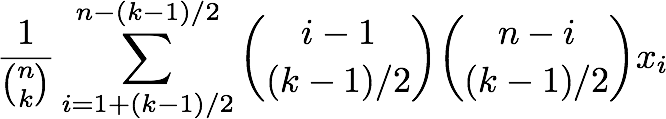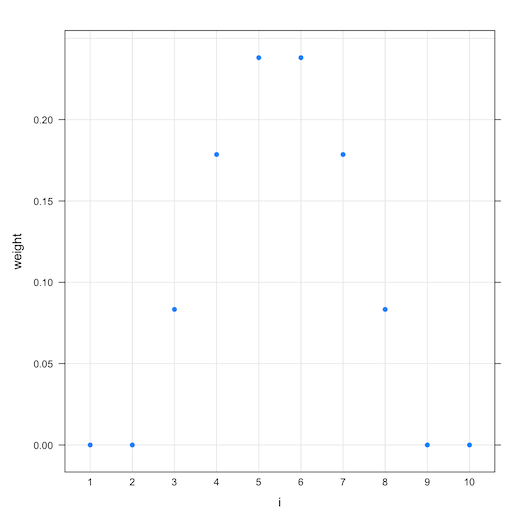# What Did Theorists Do Before The Age Of Big Data?

We have been living in the age of “big data” for some time now. This is an age where incredible things can be accomplished through the effective application of statistics and machine learning at large scale (for example see: “The Unreasonable Effectiveness of Data” Alon Halevy, Peter Norvig, Fernando Pereira, IEEE Intelligent Systems (2009)). But I have gotten to thinking about the period before this. The period before we had easy access to so much data, before most computation was aggregation and before we accepted numerical analysis style convergence as “efficient.” A small problem I needed to solve (as part of a bigger project) reminded me what theoretical computer scientists did then: we worried about provable worst case efficiency.

The problem that got me thinking is this:

Given a sequence of n integers x1 through xn and an integer k (1 ≤ k ≤ n), find the mean value of all of the medians of the k-sized selections from x1 through xn. Or as a formula:where x_s is defined as the sequence of integers whose indices are in the set s (not necessarily a contiguous sequence). The median is the “value in the middle” (a value such that half of the selected data are above it and half are below) and “(n choose k)” is the number of ways to choose k items from a collection of n items (which is just the number: n!/((n-k)! k!)). So our sum is adding up a number of terms and then dividing by the number of such terms to get the average or mean of the terms. We will call this sum a “mean of medians”.

Some obvious special cases are: for k=1 the
expression simplifies to the sum of the x_i divided by n (or just the mean of all the x_i) and for k=n it simplifies to the median of all of the x_i. For intermediate values of k it is not immediately obvious how to efficiently compute the value of the sum. Directly adding all (n choose k) terms (as the sum is written) would be very slow for large n with even moderate sized k. Instead we look to find some method that has the same value of the total sum, without directly computing all of the terms.

This gets us to the ad-hoc side of theoretical computer science. We need a clever idea. In this case the idea is simple. To keep things simple: suppose all of the n integers in our sequence have different values and that k is odd (neither of these conditions are important they just let us avoid some non-essential technicalities). What values can median(x_s) take on? median(x_s) is always x_i form some i in the subset s. In fact our sum is equivalent to:This new sum has a reasonable number of terms- so we can actually calculate it directly if we knew the values of the terms. Without loss of generality assume the x_i are sorted in increasing order. Then the number of times x_i is the median of some x_s is exactly:(and 0 for i < 1+(k-1)/2 or i > n – (k-1)/2). This is just the number of ways to place x_i in the center of a subset- by choosing (k-1)/2 smaller neighbors and (k-1)/2 larger neighbors. The count is given by multiplying the number of ways to choose smaller neighbors by the number of ways to choose the larger neighbors.

The complete solution calculating the mean of medians for distinct sorted x_i is:A statistician would recognize this expression as a kind of centrally weighted Winsorized mean. The shape of the graph of weights (in this case the n=10, k=5) is suggestive of
a bounded normal window (though i is a rank, not a free-ranging value):Likely we have re-invented a data treatment known to statisticians. But the above steps were really just combinatorics. What a theorist does is abstract something down to this sort of problem and think of variations and solutions. The formal side of theoretical computer science is attempting to organize all of the problems in the world into two classes: problems presumed easy and problems presumed hard.

For example- what if we had wanted to know the median of many means instead of the mean of many medians?
It turns out a small variation of the median of means problem is already known to be difficult. The hard version of the reversed problem is called “Kth largest subset” (this is a different K than we have been using up until now). The Kth largest subset problem is: given a sequence of integers and constants K and B do K or more distinct subsets have a sum of no more than B? The Kth largest subset problem is known to be “NP hard” which means that a whole host of other thought to be hard problems could be solved if we had the ability to solve this problem (see “Computers and Intractability: A Guide to the Theory of NP-Completeness” Michael R. Garey and David S. Johnson, 1979). The median of many means is not quite as expressive as the Kth largest subset problem (so we have not proven the median of many means is itself NP hard) but the relation is strong: to even verify that we had the right median we would need to solve a Kth largest subset problem with K=(n choose k)/2 and B= k*the_claimed_median (assuming we modified the subset problem to restrict itself to size k sub-sequences). If fact even checking if a given number is one of the possible means (let alone if it is the median of the means) is equivalent to the NP Complete knapsack problem. This correspondence makes us suspect that something as simple as the trick we devised for the mean of medians problem will not solve the median of means problem. One should not take this argument too seriously though, as the same argument would seem to apply to the pair of problems “min of means” and “mean of mins” both of which are in fact easy. We have not proven the median of means problem to be hard, we have just found relevant algorithms and related problems.

What theorists do is find these analogs and then do the heavy lifting (continue the work even when the solution is not simple) to find a way to either efficiently solve the problem at hand or prove it is in fact as hard as other important problems. This kind of thing is hard work for small gains, but the value accumulates because each of these gains is permanent. Finally additional variations of the problem are tried and characterized, to help check we hare not “leaving money on the table” (missing nearby improvements). Some of this may seem like pointless work on toy problems- but every once in a while one of these solutions or characterizations is exactly what is needed to take a large system (like a search engine) to the next level of performance.### jmount

Data Scientist and trainer at Win Vector LLC. One of the authors of Practical Data Science with R.

1.Utkarsh Samant says: